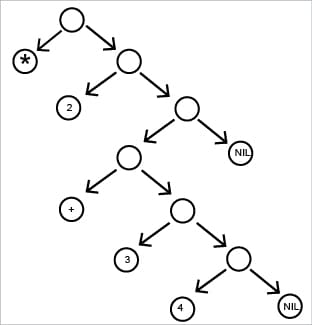# The Building Blocks of Clojure

0
1787Clojure is a high level, dynamic functional programming language. It is based on the Lisp programming language and has compilers that support Java and the .NET runtime environment. This article takes us through some of the building blocks of Clojure.

Lisp is the second-oldest high-level programming language in widespread use today. Fortran was the first, preceding Lisp by only one year. The name Lisp (earlier LISP) derives from ‘LISt Processor’. All program code in Lisp is written as s-expressions, or parenthesised lists — it is functional programming that supports homoiconicity.

S-expressions, sexprs or sexps (for ‘symbolic expression’ ) are a notation for nested list (tree-structured) data, invented for the programming language Lisp, which uses them for source code as well as data. An s-expression is classically defined as an expression of the form (x . y) where x and y are s-expressions.

The recursive part of the definition represents an ordered pair, which means that s-expressions are binary trees. As an example, (x y z) stands for (x . (y . (z . NIL))), where NIL is the special end-of-list object. You can visit the wiki page at https://en.wikipedia.org/wiki/S-expression for more information on this.In the above binary tree, if we use the ‘in-order’ tree traversal, we could derive the mathematical expression as:

( * 2 ( + 3 4 ) ).

Functional programming is a programming paradigm—a style of building the structure and elements of computer programs that treat computation as the evaluation of mathematical functions and avoids changing-state and mutable data.

The key principle that functional programming is based on is that all computation is the execution of a series of mathematical functions. And hence the term ‘functional programming’.

```• function: mapping from input to output
F(x) = x * 5
x -> could be 1, 2.. n
G(x,y) = F(x)+ F(y)
G(10,1) = F(10) + F(1)
10*5 + 1*5
55
H(x) = {1, if x is even; 0, if x is odd}```
• These mathematical functions can’t change their input.
• And they can’t change the variable that displays immutability.

If we can’t change the variable value then we cannot use the operations given below:

```x = x+1 and
for (i=0; i<10; i++)```

But Clojure provides beautiful techniques to achieve that without changing the variable.

Programming without variable assignment
Homoiconicity (from the Greek words homo, which means ‘the same’, and ‘icon’, which means ‘representation’) is a property of some programming languages. A language is homoiconic if a program written in it can be manipulated as data using the language, and thus the program’s internal representation can be inferred just by reading the program itself.

For example, a Lisp program is written as a regular Lisp list and can be manipulated by other Lisp code. In homoiconic languages, all code can be accessed and transformed as data, using the same representation. This property is often summarised by saying that the language treats ‘code as data’.

Installation
There are a variety of ways to work with Clojure as a programming language.

• Leiningen: This is an essential tool to create, build and automate Clojure projects.
• Eclipse plugin: There is a plugin called CounterClockwise, which is available to carry out Clojure development in the Eclipse IDE.